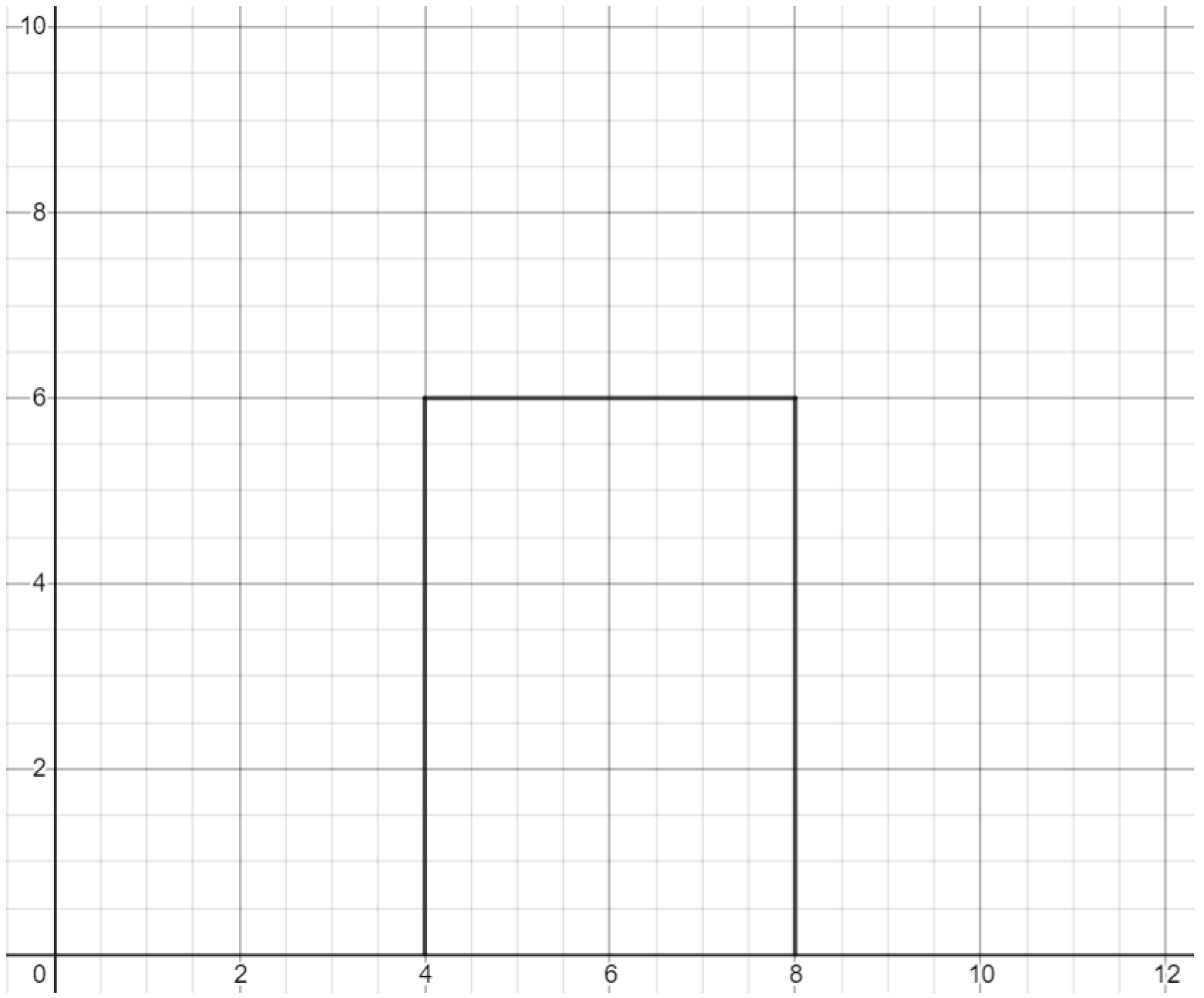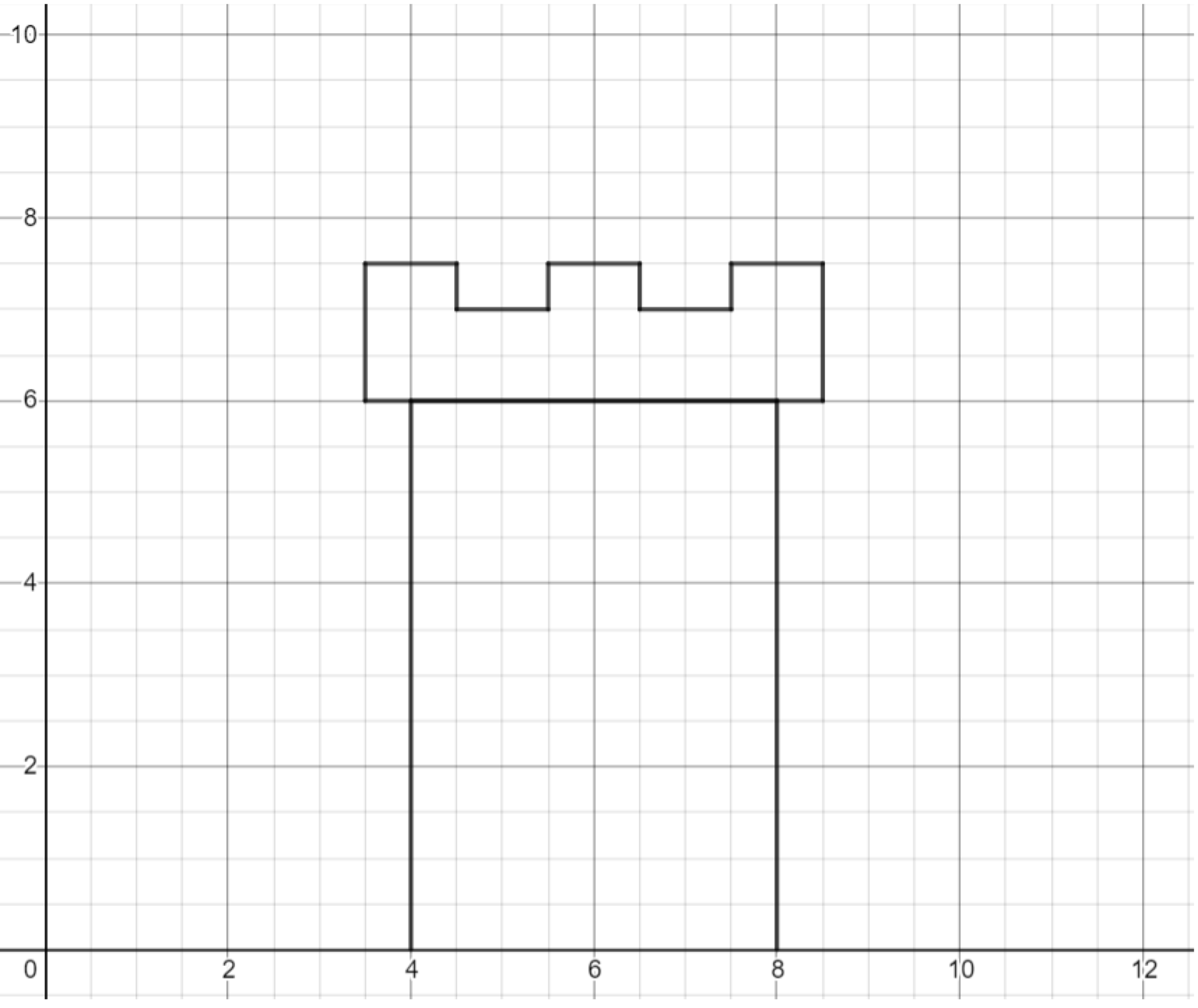# Graphing Calculator Drawing: Horizontal and Vertical Lines

This post is part of a series.

Demonstration - Vertical Lines. Observe the graph as you type each of the following inputs:

\begin{align*} x &= 1 \\[10pt] x &= 2 \\[10pt] x &= 3 \end{align*}

Demonstration - Horizontal Lines. Observe the graph as you type each of the following inputs:

\begin{align*} y &= 1 \\[10pt] y &= 2 \\[10pt] y &= 3 \end{align*}

Demonstration - Rays and Segments. Observe the graph as you type each of the following inputs:

\begin{align*} x&=1 \lbrace 2 < y \rbrace \\[10pt] x&=2 \lbrace 2 < y < 3 \rbrace \\[10pt] y&=1 \lbrace x < 2 \rbrace \\[10pt] y&=2 \lbrace 0 < x < 2 \rbrace \end{align*}

Exercise. Reproduce the graph below. (Note: you can change the line color by clicking and holding the colored circle to the left of the math input box.)Exercise. Add ridges to the top of the graph you produced in the previous example.Challenge. Draw a full castle!

This post is part of a series.

Tags: